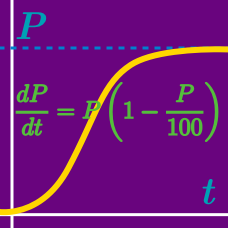Calculus

# Differential Equations - dy/dx = g(y)

If $\displaystyle \frac{dy}{dx}=9x^2y$ and $y(0)=6,$ which of the following is true?

If $\displaystyle \frac{dy}{dx}=\frac{8x}{y}$ and $y(0)=3,$ which of the following is true?

If $\displaystyle \frac{dy}{dx}=\frac{y^2}{x}$ and $\displaystyle y(1)=\frac{1}{8},$ which of the following is true?

If $\displaystyle \frac{dy}{dx}=e^y$ and $y=0$ when $x=6,$ which of the following is true?

If $\displaystyle \frac{dy}{dx}=8xy^2$ and $\displaystyle y(0)=\frac{1}{5},$ which of the following is true?

×